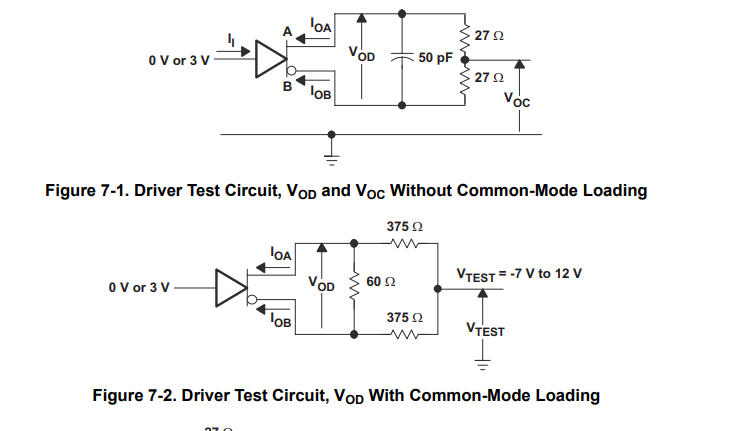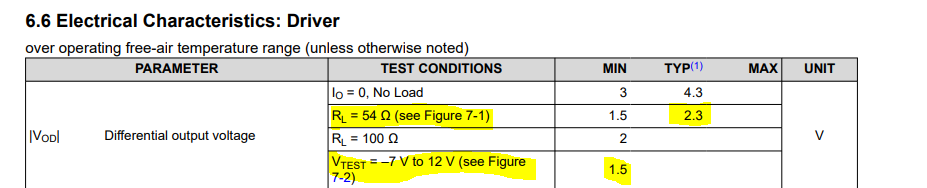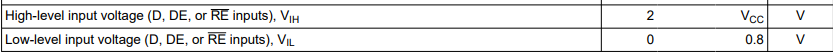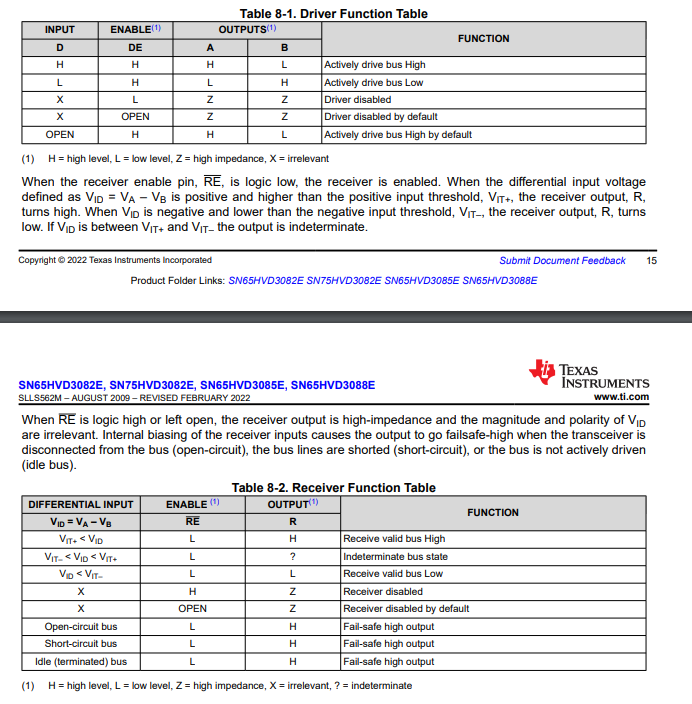If you have a related question, please click the "Ask a related question" button in the top right corner. The newly created question will be automatically linked to this question.

# SN65HVD3082E: For the respective output voltages of Apin and Bpin

Part Number: SN65HVD3082E

Hi team,

My customer is trying to verify the Apin and Bpin voltages in the final test.

However, the datasheet only describes differential voltage.
Since RS485 is a differential voltage, could you tell me how many volts it should be at (A-B) to be as per the datasheet?

Please tell me what voltage would be for each combination for  Dpin and DEpin?

The termination resistor is 120 Ω between A and B near the device.

Sincerely.
Kengo.

• The RS-485 specification requires a voltage drop of at least 1.5 V over a bus with two 120 Ω termination resistors, one at each end. (60 Ω − 10 % gives you the RL = 54 Ω test condition in the electrical characteristics.)

For the behaviour of the D and DE pins, see table 8-1.

• Hi Kengo,

Clemens is correct on the minimum (under rated conditions) be 1.5V with two 120 Ohm termination resistors between A and B (on each extreme end of the bus).

For this device specifically:

The test setups look like the following:With the corresponding data:So if you use a setup similar to 7-1 (even without the capacitance - as that shouldn't effect the steady-state Vod voltage too much) you should get ~2.3V for room temperature standard setup conditions (it doesn't need to be exactly 2.3V but it should be pretty close) but it shouldn't be lower than 1.5V. If common mode loading is tested we only have a min of 1.5V rated. We also have tests with a 100 Ohm termination (For RS-422 standard) and a no load output voltage (which should be pretty close to VCC (with a couple diode drops)).

The logic thresholds for D, DE, and /RE are the same and shown below:With the truth tables shown below:All this information can be found in the datasheet for future reference.

Please let me know if there are any other questions!

Best,

Parker Dodson

• Hi Clemens, Paler,

If there is more than 1.5V at each of the Apin and Bpin, does that mean there is no problem?

The following conditions were verified by my customer.

＜DEpin : H、Dpin :  H＞
Apin：3.94V
Bpin：1.19V

Since the B terminal is a Low output, does this mean that the result is lower than 1.5V?

Sincerely.
Kengo.

• Anything above 1.5 V is OK. You are getting 2.75 V, so I guess your load is larger than 54 Ω.

The differential output voltage might be positive or negative, which is why |VOD| is specified as the absolute value.